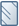## Blog Pagination Showing Page 0

SOLVE
New Contributor

A client's live Blog allows you to visit www.url.com/blog/page/0 which is generating a lot of broken links. How do I set it so it the lowest page number is 1?

1 Accepted solution

Accepted Solutions
Highlighted
JanetArmstrongRegular Contributor | Silver Partner

If you have old blog posts with broken links you should fix them or delete.

What does the code for blog listing in your template read?  This is what mine has:

``` <div class="blog-pagination">
{% if last_page_num %}
{% endif %}
<a class="hs-button primary all-posts-link" href="{{ group.absolute_url }}/all">All posts</a>
{% if next_page_num %}
{% endif %}
</div>```
3 Replies 3
Highlighted
JanetArmstrongRegular Contributor | Silver Partner

If you have old blog posts with broken links you should fix them or delete.

What does the code for blog listing in your template read?  This is what mine has:

``` <div class="blog-pagination">
{% if last_page_num %}
{% endif %}
<a class="hs-button primary all-posts-link" href="{{ group.absolute_url }}/all">All posts</a>
{% if next_page_num %}
{% endif %}
</div>```
New Contributor

{% set total_pages = contents.total_page_count %} <!-- sets variable for total pages -->
{% set more_pages = total_pages - current_page_num %} <!-- sets variable for how many more pages are past the current pages -->

{% if total_pages > 0 %}
<div class="pagination-main">
<span class="{% if current_page_num == 1 %}disabled{% endif %}"><a href="{% if last_page_num=="1" %}{{ group.absolute_url }}{% else %}{{blog_page_link(last_page_num)}}{% endif %}" class="previous-link"><i class="fa fa-angle-left"></i></a></span>
<ul class="blog-pagination">

{% if more_pages == 1 %}
{% if current_page_num - 4 == 1 %}<li><a href="{{ group.absolute_url }}">{{ current_page_num - 4 }}</a></li>{% endif %}
{% if current_page_num - 4 > 1 %}<li><a href="{{ blog_page_link(current_page_num - 4) }}">{{ current_page_num - 4 }}</a></li>{% endif %}
{% endif %}
{% if more_pages <= 1 %}
{% if current_page_num - 3 == 1 %}<li><a href="{{ group.absolute_url }}">{{ current_page_num - 3 }}</a></li>{% endif %}
{% if current_page_num - 3 > 1 %}<li><a href="{{ blog_page_link(current_page_num - 3) }}">{{ current_page_num - 3 }}</a></li>{% endif %}
{% endif %}
{% if current_page_num - 2 == 1 %}<li><a href="{{ group.absolute_url }}">{{ current_page_num - 2 }}</a></li>{% endif %}
{% if current_page_num - 2 > 1 %}<li><a href="{{ blog_page_link(current_page_num - 2) }}">{{ current_page_num - 2 }}</a></li>{% endif %}
{% if current_page_num - 1 == 1 %}<li><a href="{{ group.absolute_url }}">{{ current_page_num - 1 }}</a></li>{% endif %}
{% if current_page_num - 1 > 1 %}<li><a href="{{ blog_page_link(current_page_num - 1) }}">{{ current_page_num - 1 }}</a></li>{% endif %}
<li class="active"><a href="{% if current_page_num==1 %}{{ group.absolute_url }}{% else %}{{ blog_page_link(current_page_num) }}{% endif %}">{{ current_page_num }}</a></li>
{% if current_page_num + 1 <= total_pages %}<li><a href="{{ blog_page_link(current_page_num + 1) }}">{{ current_page_num + 1 }}</a></li>{% endif %}
{% if current_page_num + 2 <= total_pages %}<li><a href="{{ blog_page_link(current_page_num + 2) }}">{{ current_page_num + 2 }}</a></li>{% endif %}
{% if current_page_num <= 2 %}
{% if current_page_num + 3 <= total_pages %}<li><a href="{{ blog_page_link(current_page_num + 3) }}">{{ current_page_num + 3 }}</a></li>{% endif %}
{% endif %}
{% if current_page_num == 1 %}
{% if current_page_num + 4 <= total_pages %}<li><a href="{{ blog_page_link(current_page_num + 4) }}">{{ current_page_num + 4 }}</a></li>{% endif %}
{% endif %}
</ul>
{% if total_pages >= current_page_num %}
<span class="{% if current_page_num == total_pages %}disabled{% endif %}"><a href="{{blog_page_link(next_page_num)}}" class="next-link"><i class="fa fa-angle-right"></i></a></span>
{% endif %}
</div>
{% endif %}
</div>
</div>

JanetArmstrongRegular Contributor | Silver Partner

You can replace that with what I showed you above.